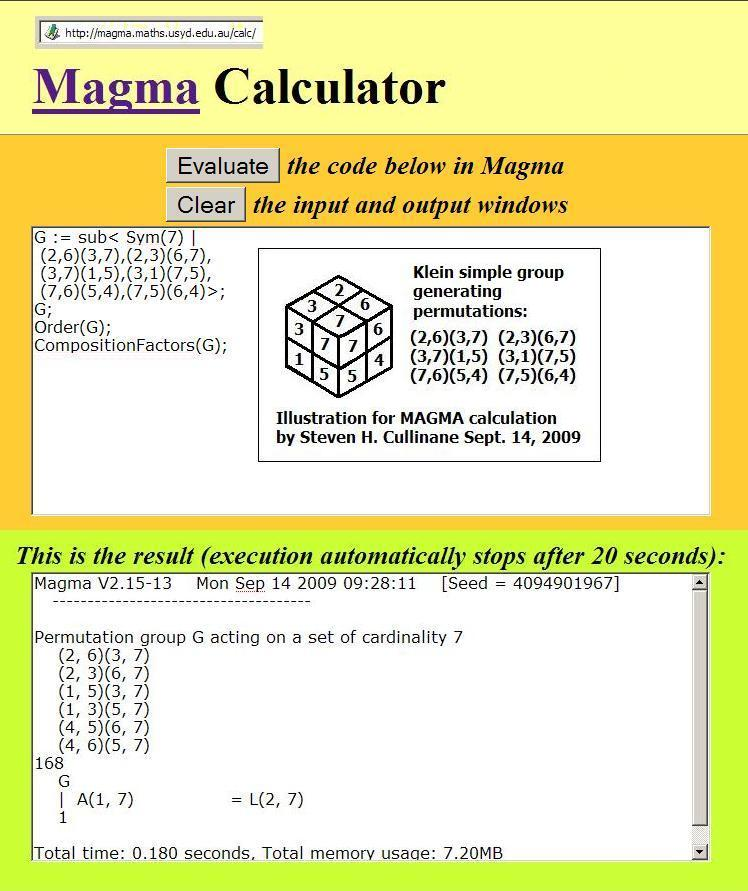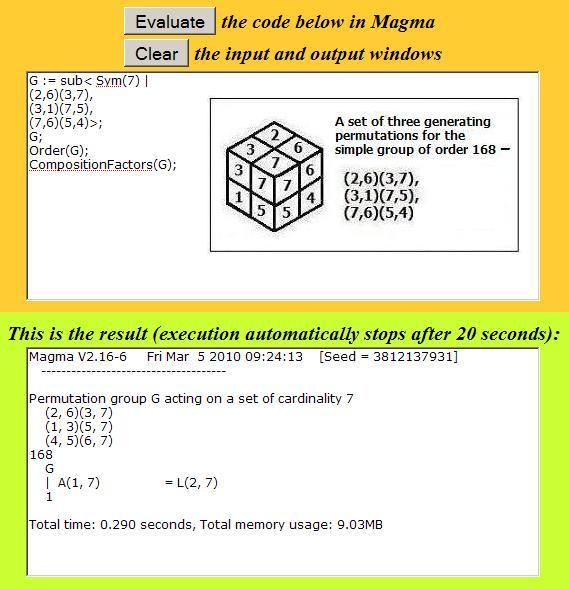Finite Geometry Notes   | Home | Site Map | Author |

Calculating an Order-168
Reflection Group

by Steven H. Cullinane Sept. 14, 2009

The image below is an illustration for
"A Reflection Group of Order 168"
and "The Eightfold Cube."

The calculation indicates that Klein's
simple group of order 168 is generated
by permuting parallel 1x1x2 "bricks" in
each of three halves of a 2x2x2 cube.
Each such permutation is a reflection:
a linear transformation of a vector space
that fixes a hyperplane pointwise.Here "A(1, 7)" is a MAGMA designation
for the group L2(7) (Atlas notation),
which is isomorphic to the group L3(2).
The group action illustrated by the permutations
in the calculation is of course that of L3(2).

Note of March 4, 2010:

The group L3(2) can be generated by using
only three of the six permutations above--